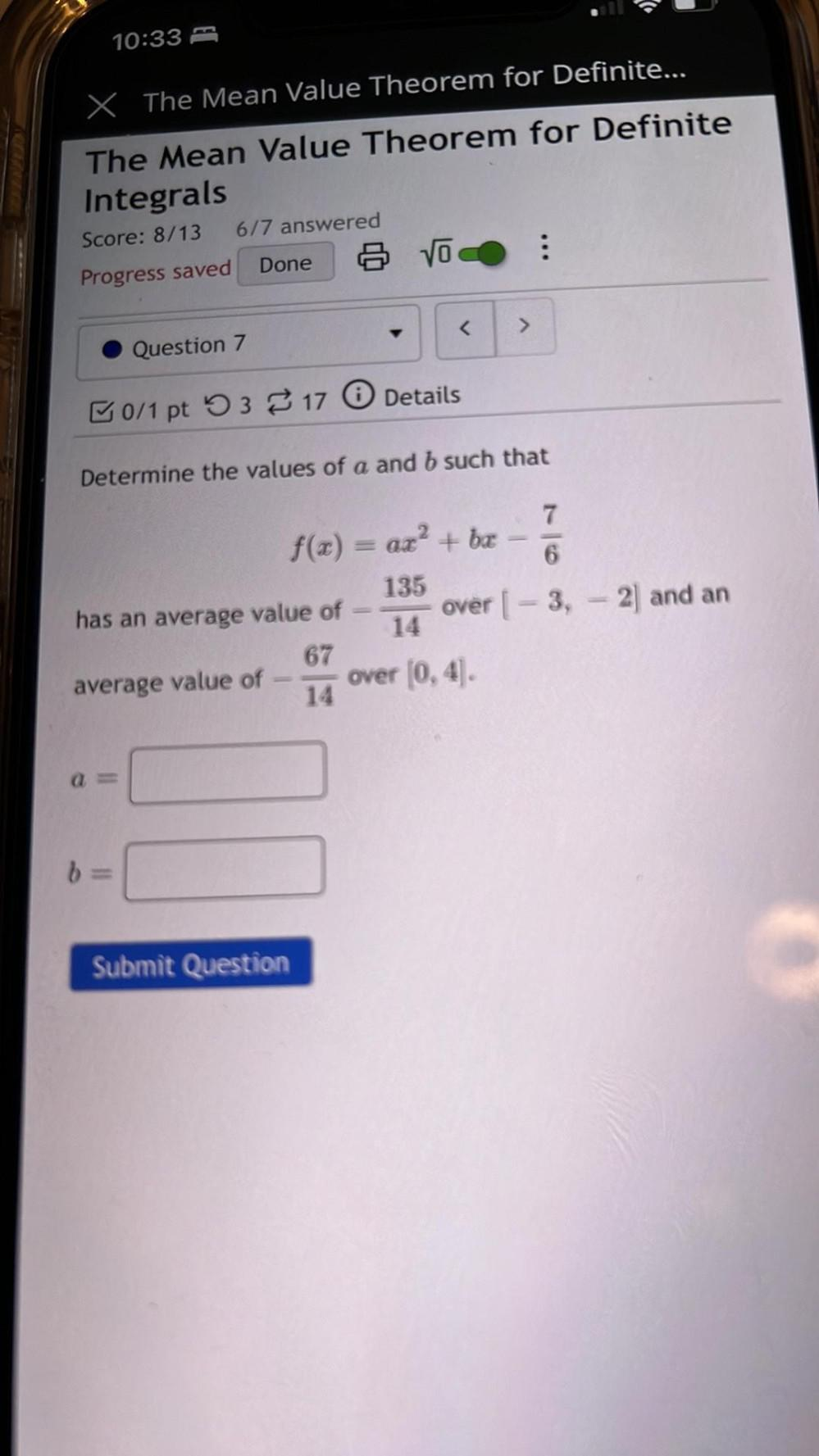Question:

# 10:33 x The Mean Value Theorem for Definite... The Mean Value Theorem for Definite Integrals Score: 8/13 6/7 answered Progress s10:33 x The Mean Value Theorem for Definite... The Mean Value Theorem for Definite Integrals Score: 8/13 6/7 answered Progress saved 6 VO : Done < > Question 7 B0/1 pt 93 317 0 Details Determine the values of a and b such that 7 f(x) = ax? + bæ 6 135 has an average value of over [ – 3, - 2) and an 14 67 average value of over (0,4). 14 L b Submit Question